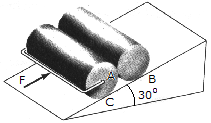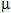# Engineering Mechanics - Friction - Discussion

### Discussion :: Friction - General Questions (Q.No.3)

3.Determine the minimum force F needed to push the two 75-kg cylinders up the incline. The force acts parallel to the plane and the coefficients of friction at the contacting surfaces areA = 0.3,B = 0.25,C = 0.4. Each cylinder has a radius of 150 mm.

 [A]. F = 919 N [B]. F = 735 N [C]. F = 1.051 kN [D]. F = 981 N

Explanation:

No answer description available for this question.

 Vemula Naresh said: (Sep 7, 2012) Draw the fbd for given block , consider that two block as one body and write the vector components weight downwards, friction opposite to the applied force F . and indicate the normal reaction to the block . First of all find the normal reaction by summation of forces along normal line . we will get normal . multiply normal with static frictional coefficient you will be able to get frictional force. Take summation of forces along the inclined plane you will get the applied force F.